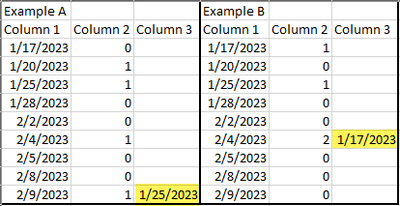cancel
Showing results for
Did you mean:Frequent Visitor

## Getting a specific date value based on another columns value

Hello all,

First time poster, long time reader.
I have a set of data that I need to get a specific date out of and I am just not getting what I need. Here is a basic snippit of the data:What I need to do is starting from the most recent date where there is a 1 (or higher) find the third to last date where there is also a 1 (or higher). In Example A, since the most recent date is 2/9/23 it should return 1/25/2023. In Example B, the most recent date is 2/4/2023 then it would be 1/17/2023. So the result should look like this:On top of that, I need to advance that date forward by 90 days so the end result would be 4/25/2023 and 4/17/2023, respectively.
Eventually I will only show the final date result in my visual and I'm sure I can figure that out, I just can't seem to get any DAX to work to give me the result I need. I have tried TOPN, RANKX, Among others and I would imagine that my inexperience, I have only used DAX a handful of times, is the reason I am not getting this correct.

TIA

1 ACCEPTED SOLUTIONCommunity Champion
Sorry i misconsidered that last point. try like:
Measure =
VAR _table=
CALCULATETABLE(
VALUES(TableName[Col1]),
TableName[Col2]=1
)
VAR _top3 =
TOPN(3, _table, TableName[Col1] )
VAR _top2=
TOPN(2, _table, TableName[Col1] )
RETURN
EDATE(EXCEPT(_top3, _top2), 3)
8 REPLIES 8Solution Specialist

Hi @MikeDubya ,

I think the function you are looking for is the brand new "OFFSET" function.

OFFSET – DAX GuideCommunity Champion

try to plot a measure like:

Measure =
VAR _table=
CALCULATETABLE(
VALUES(TableName[Col1]),
TableName[Col2]=1
)
VAR _top3 =
TOPN(3, _table, TableName[Col1] )
VAR _top2=
TOPN(2, _table, TableName[Col1] )
RETURN
EXCEPT(_top3, _top2)Frequent Visitor

This works great! The only thing left is to add 90 days to the result that the above gives. I set the "EXCEPT(_top3, _top2)" to a variable called final ( which worked fine if I just put final after RETURN) and then tried DATEADD(final, 90, DAY) but it just gave me a blank result. Thoughts? Should I add the 90 to another variable?Community Champion
Sorry i misconsidered that last point. try like:
Measure =
VAR _table=
CALCULATETABLE(
VALUES(TableName[Col1]),
TableName[Col2]=1
)
VAR _top3 =
TOPN(3, _table, TableName[Col1] )
VAR _top2=
TOPN(2, _table, TableName[Col1] )
RETURN
EDATE(EXCEPT(_top3, _top2), 3)Frequent Visitor

That's it! Thank you so much!Community Champion

this works mainly because one-row-one-column table can be treated as value in DAX directly.Frequent Visitor

I did have one more question. There is a bit of a change to what I need. In example B, you'll notice that instead of a 1 there is a 2 on one of the dates. This will happen often in the real data. I didn't know this when I originally posted but the total of the column should count/add to 3 and whereever the third is counted that is the date we want. So for example B, the date that should be returned is 1/25/2023 not 1/17/2023 because the second column, from most recent date to older, would add up to 3 there. The first example is still correct. Any thoughts? I dinked around with the code you have but not sure where to have it count that. I'd imagine an IF statement would handle this but curious what you think. To make it clear, once the total of the second column is 3 or greater, what is the date where it hits 3?Frequent Visitor

For more context, I am part of a group of people that fly and we want to see if power bi can calculate our currency. So if the dates are when I or someone else flew and the 0, 1, 2, or more is say how many times we took off, our currency is calculated from the third to last take off in this case. Sometimes we fly more than once a day so that needs to be calculated into the result.